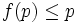# Minimal operator

This article defines a subgroup property modifier (a unary subgroup property operator) -- viz an operator that takes as input a subgroup property and outputs a subgroup property

View a complete list of subgroup property modifiers OR View a list of all subgroup property operators (possibly with multiple inputs)

## Definition

The minimal operator is a subgroup property modifier that takes as input a subgroup property$p$ and outputs a subgroup property$q$ defined as follows:$H$ has property$q$ in$G$ if the following three hold:

•$H$ has property$p$ in$G$
•$H$is a nontrivial subgroup of$G$
• There is no nontrivial subgroup$K$ of$G$, contained in$H$, such that$K$ satisfies$p$.

In other words,$H$ is a minimal element (in the containment partial order) among those nontrivial subgroups of$G$ that satisfy$p$.

## Application

Some important instances of application of the maximal operator:

• minimal normal subgroup: obtained from normal subgroup
• minimal characteristic subgroup: obtained from characteristic subgroup

## Properties

### Monotonicity

The minimal operator is not monotone. In other words, if$p \le q$ are subgroup properties, then a maximal$p$-subgroup need not be a maximal$q$-subgroup. The reason is that there may be smaller subgroups that satisfy property$q$ but not property$p$.

### Descendance

This subgroup property modifier is descendant, viz the image of any subgroup property under this modifier is stronger than that property. In symbols, if$f$ denotes the modifier and$p$ and property,$f(p) \le p$

For any subgroup property$p$, the property of being a minimal$p$-subgroup, is stronger than the property of being a$p$-subgroup.

### Idempotence

This subgroup property modifier is idempotent, viz applying it twice to a subgroup property has the same effect as applying it once

Clearly, applying the maximal operator twice has the same effect as applying it once. Those subgroup properties that are obtained by applying this operator are termed minimal subgroup properties.